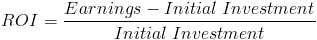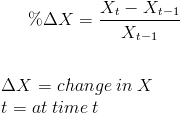# Return on Investment

ROI Calculator (Click Here or Scroll Down)The formula for return on investment, sometimes referred to as ROI or rate of return, measures the percentage return on a particular investment. ROI is used to measure profitability for a given amount of time.

The return on investment formula is mechanically similar to other rate of change formulas, an example being rate of inflation. The base formula for measuring a percentage rate of change is:For ROI, we are measuring the rate of change of monies due to investing. By applying the return on investment formula, we can determine a X% change in monies on an investment, which is synonymous with a X% return on investment.

## Use of ROI Formula

The return on investment formula is used loosely in finance and investing. It can be applied to any form of investment including projects within a corporation, a company as a whole, a personal investment by an individual, and investment in an appreciable asset.

Other similar formulas that measure profitability are return on equity, return on assets, and return on capital, among others.

One issue with the return on investment formula is that it does not account for the time value of money. This issue is not entirely detrimental if one considers to account for the time value of money, post facto. The "earnings" portion of the formula could also be discounted to the present value at the origin of the project or investment. This, however, can become very tricky if the costs occur at different time periods. At times, the net present value method may be preferred due to these reasons.

New to Finance?